# A buffer solution contains 0.229 M ammonium chloride and 0.457 M ammonia. If 0.0568 moles of...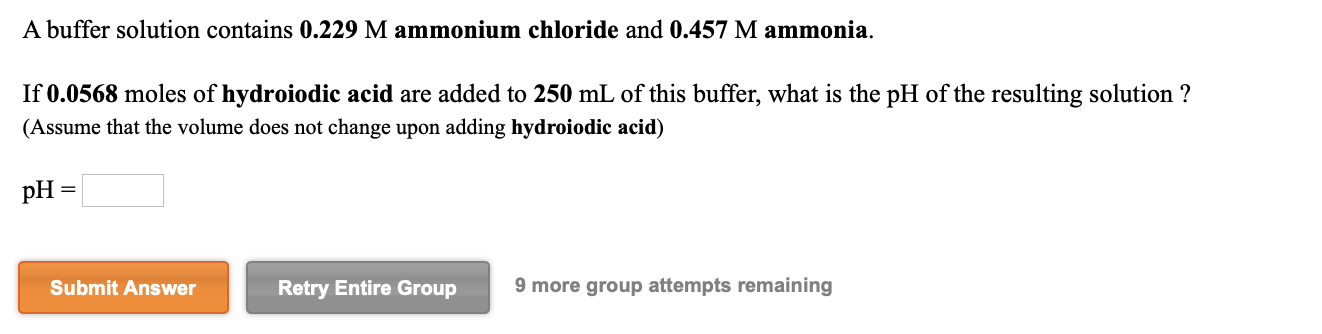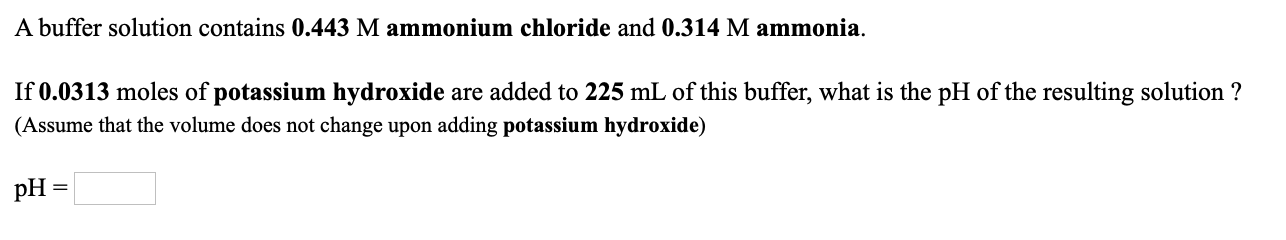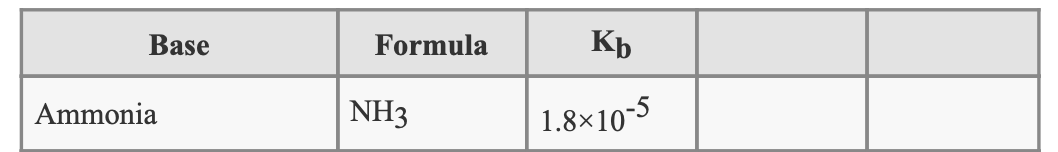A buffer solution contains 0.229 M ammonium chloride and 0.457 M ammonia. If 0.0568 moles of hydroiodic acid are added to 250 mL of this buffer, what is the pH of the resulting solution ? (Assume that the volume does not change upon adding hydroiodic acid) pH = Submit Answer Retry Entire Group 9 more group attempts remaining
A buffer solution contains 0.443 M ammonium chloride and 0.314 M ammonia. If 0.0313 moles of potassium hydroxide are added to 225 mL of this buffer, what is the pH of the resulting solution ? (Assume that the volume does not change upon adding potassium hydroxide) pH =
Base Formula Кь Ammonia NH3 1.8x10-5

1)

mol of HI added = 0.0568 mol

NH3 will react with H+ to form NH4+

Before Reaction:
mol of NH3 = 0.457 M *0.25 L
mol of NH3 = 0.1143 mol

mol of NH4+ = 0.229 M *0.25 L
mol of NH4+ = 0.0573 mol

after reaction,
mol of NH3 = mol present initially - mol added
mol of NH3 = (0.1143 - 0.0568) mol
mol of NH3 = 0.0575 mol

mol of NH4+ = mol present initially + mol added
mol of NH4+ = (0.0573 + 0.0568) mol
mol of NH4+ = 0.1141 mol
since volume is both in numerator and denominator, we can use mol instead of concentration
Kb = 1.8*10^-5

pKb = - log (Kb)
= - log(1.8*10^-5)
= 4.745

use:
pOH = pKb + log {[conjugate acid]/[base]}
= 4.745+ log {0.1141/5.745*10^-2}
= 5.043

use:
PH = 14 - pOH
= 14 - 5.0425
= 8.9575

2)

mol of KOH added = 0.0313 mol

NH4+ will react with OH- to form NH3

Before Reaction:
mol of NH3 = 0.443 M *0.225 L
mol of NH3 = 0.0997 mol

mol of NH4+ = 0.314 M *0.225 L
mol of NH4+ = 0.0707 mol

after reaction,
mol of NH3 = mol present initially + mol added
mol of NH3 = (0.0997 + 0.0313) mol
mol of NH3 = 0.131 mol

mol of NH4+ = mol present initially - mol added
mol of NH4+ = (0.0707 - 0.0313) mol
mol of NH4+ = 0.0394 mol
since volume is both in numerator and denominator, we can use mol instead of concentration
Kb = 1.8*10^-5

pKb = - log (Kb)
= - log(1.8*10^-5)
= 4.745

use:
pOH = pKb + log {[conjugate acid]/[base]}
= 4.745+ log {3.935*10^-2/0.131}
= 4.222

use:
PH = 14 - pOH
= 14 - 4.2225
= 9.7775

#### Earn Coin

Coins can be redeemed for fabulous gifts.

Similar Homework Help Questions
• ### A buffer solution contains 0.341 M ammonium chloride and 0.291 M ammonia. If 0.0213 moles of potassium hydroxide are add...

A buffer solution contains 0.341 M ammonium chloride and 0.291 M ammonia. If 0.0213 moles of potassium hydroxide are added to 150 mL of this buffer, what is the pH of the resulting solution ? (Assume that the volume does not change upon adding potassium hydroxide) pH=?

• ### A buffer solution contains 0.459 M ammonium bromide and 0.226 M ammonia. If 0.0482 moles of...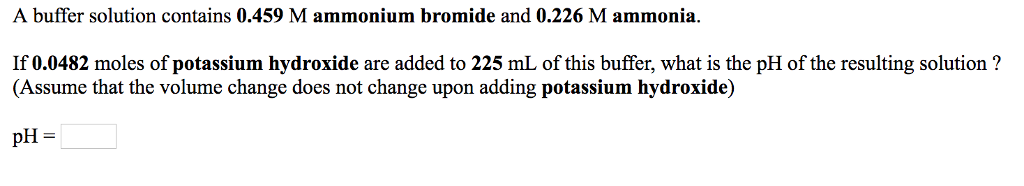A buffer solution contains 0.459 M ammonium bromide and 0.226 M ammonia. If 0.0482 moles of potassium hydroxide are added to 225 mL of this buffer, what is the pH of the resulting solution? (Assume that the volume change does not change upon adding potassium hydroxide) H=

• ### A buffer solution contains 0.392 M ammonium chloride and 0.498 M ammonia. If 0.0206 moles of...

A buffer solution contains 0.392 M ammonium chloride and 0.498 M ammonia. If 0.0206 moles of perchloric acid are added to 150 mL of this buffer, what is the pH of the resulting solution ? (Assume that the volume does not change upon adding perchloric acid)

• ### 1. A buffer solution contains 0.256 M hydrofluoric acid and 0.308 M potassium fluoride. If 0.0414...

1. A buffer solution contains 0.256 M hydrofluoric acid and 0.308 M potassium fluoride. If 0.0414 moles of hydrochloric acid are added to 225 mL of this buffer, what is the pH of the resulting solution ? (Assume that the volume does not change upon adding hydrochloric acid) pH = 2. A buffer solution contains 0.328 M ammonium chloride and 0.331 M ammonia. If 0.0396 moles of sodium hydroxide are added to 225 mL of this buffer, what is the...

• ### A buffer solution contains 0.225 M ammonium bromide and 0.318 M ammonia, If 0.0153 moles of...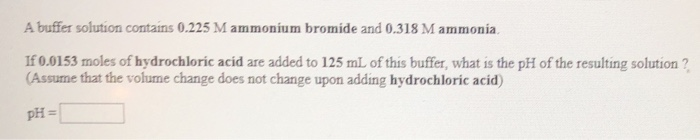A buffer solution contains 0.225 M ammonium bromide and 0.318 M ammonia, If 0.0153 moles of hydrochloric acid are added to 125 mL of this buffer, what is the pH of the resulting solution? (Assume that the volume change does not change upon adding hydrochloric acid) pH =

• ### A buffer solution contains 0.207 M NaHSO3 and 0.428 M Na S03. If 0.0299 moles of...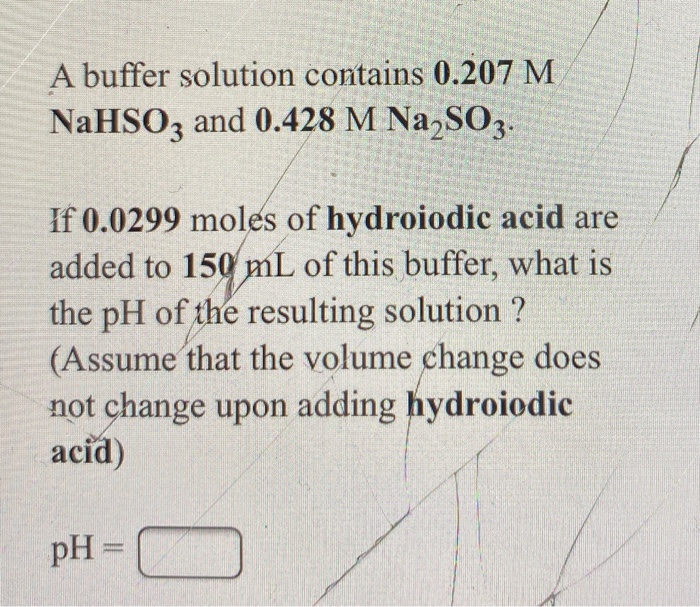A buffer solution contains 0.207 M NaHSO3 and 0.428 M Na S03. If 0.0299 moles of hydroiodic acid are added to 150 mL of this buffer, what is the pH of the resulting solution ? (Assume that the volume change does not change upon adding hydroiodic acid) pH = 1

• ### If anymore values are needed let me know A buffer solution contains 0.378 M KH2PO4 and...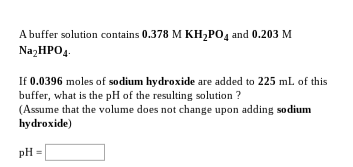If anymore values are needed let me know A buffer solution contains 0.378 M KH2PO4 and 0.203 M NagHPOд If 0.0396 moles of sodium hydroxide are added to 225 mL of this buffer, what is the pH of the resulting solution? (Assume that the volume does not change upon adding sodium hydroxide) pH A buffer solution contains 0.420 M ammonium chloride and 0.389 M ammonia If 0.0191 moles of potassium hydroxide are added to 150 mL of this buffer, what...

• ### A 1.00 liter solution contains 0.34 M ammonia and 0.44 M ammonium bromide. If 0.110 moles...

A 1.00 liter solution contains 0.34 M ammonia and 0.44 M ammonium bromide. If 0.110 moles of barium hydroxide are added to this system, indicate whether the following statements are true or false. (Assume that the volume does not change upon the addition of barium hydroxide.) _______TrueFalse A. The number of moles of NH3 will increase. _______TrueFalse B. The number of moles of NH4+ will remain the same. _______TrueFalse C. The equilibrium concentration of H3O+ will remain the same. _______TrueFalse...

• ### What is the pH of a buffer prepared by mixing 20.00 mL of 0.0300 M ammonium chloride with 40.00 mL of 0.0450 M ammonia?...

What is the pH of a buffer prepared by mixing 20.00 mL of 0.0300 M ammonium chloride with 40.00 mL of 0.0450 M ammonia? What is the resulting pH if 1.00 mL of 0.10 M HCl is added to this solution?

• ### A 1.00 liter solution contains 0.36 M ammonia and 0.47 M ammonium bromide. If 0.240 moles...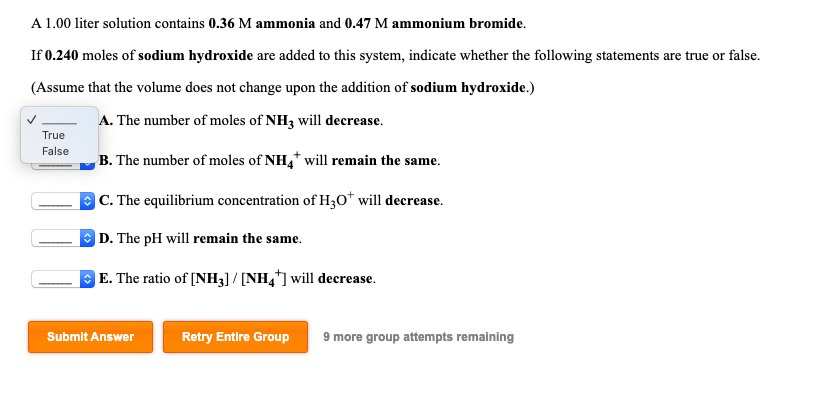A 1.00 liter solution contains 0.36 M ammonia and 0.47 M ammonium bromide. If 0.240 moles of sodium hydroxide are added to this system, indicate whether the following statements are true or false. (Assume that the volume does not change upon the addition of sodium hydroxide.) A. The number of moles of NH3 will decrease. True False B. The number of moles of NH will remain the same. C. The equilibrium concentration of H30* will decrease. D. The pH will...

Free Homework App